In elementary algebra, a quadratic equation (from the Latin quadratus for "square") is any equation having the formwhere x represents an unknown, and a, b, and c represent known numbers such that a is not equal to 0. If a = 0, then the equation is linear, not quadratic. The numbers a, b, and c are the coefficients of the equation, and may be distinguished by calling them, respectively, the quadratic coefficient, the linear coefficient and the constant or free term.

Because the quadratic equation involves only one unknown, it is called "univariate". The quadratic equation only contains powers of x that are non-negative integers, and therefore it is a polynomial equation, and in particular it is a second degree polynomial equation since the greatest power is two.

Quadratic equations can be solved by a process known in American English as factoring and in other varieties of English as factorising, by completing the square, by using the quadratic formula, or by graphing. Solutions to problems equivalent to the quadratic equation were known as early as 2000 BC.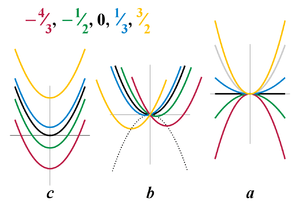Figure 1. Plots of quadratic function y = ax2 + bx + c, varying each coefficient separately while the other coefficients are fixed (at values a = 1, b = 0, c = 0)

A quadratic equation with real or complex coefficients has two solutions, called roots. These two solutions may or may not be distinct, and they may or may not be real.

### Factoring by inspection

It may be possible to express a quadratic equation ax2 + bx + c = 0 as a product (px + q)(rx + s) = 0. In some cases, it is possible, by simple inspection, to determine values of p, q, r, and s that make the two forms equivalent to one another. If the quadratic equation is written in the second form, then the "Zero Factor Property" states that the quadratic equation is satisfied if px + q = 0 or rx + s = 0. Solving these two linear equations provides the roots of the quadratic.

For most students, factoring by inspection is the first method of solving quadratic equations to which they are exposed.:202207 If one is given a quadratic equation in the form x2 + bx + c = 0, the sought factorization has the form (x + q)(x + s), and one has to find two numbers q and s that add up to b and whose product is c (this is sometimes called "Vieta's rule" and is related to Vieta's formulas). As an example, x2 + 5x + 6 factors as (x + 3)(x + 2). The more general case where a does not equal 1 can require a considerable effort in trial and error guess-and-check, assuming that it can be factored at all by inspection.

Except for special cases such as where b = 0 or c = 0, factoring by inspection only works for quadratic equations that have rational roots. This means that the great majority of quadratic equations that arise in practical applications cannot be solved by factoring by inspection.:207

### Completing the square

Main article: Completing the square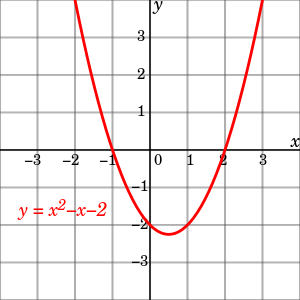Figure 2. For the quadratic function y = x2 x 2, the points where the graph crosses the x-axis, x = −1 and x = 2, are the solutions of the quadratic equation x2 x 2 = 0.

The process of completing the square makes use of the algebraic identitywhich represents a well-defined algorithm that can be used to solve any quadratic equation.:207 Starting with a quadratic equation in standard form, ax2 + bx + c = 0

1. Divide each side by a, the coefficient of the squared term.
2. Subtract the constant term c/a from both sides.
3. Add the square of one-half of b/a, the coefficient of x, to both sides. This "completes the square", converting the left side into a perfect square.
4. Write the left side as a square and simplify the right side if necessary.
5. Produce two linear equations by equating the square root of the left side with the positive and negative square roots of the right side.
6. Solve the two linear equations.

We illustrate use of this algorithm by solving 2x2 + 4x 4 = 0The plus-minus symbol "±" indicates that both x = 1 + 3 and x = 1 3 are solutions of the quadratic equation.

### Quadratic formula and its derivation

Completing the square can be used to derive a general formula for solving quadratic equations, called the quadratic formula. The mathematical proof will now be briefly summarized. It can easily be seen, by polynomial expansion, that the following equation is equivalent to the quadratic equation:Taking the square root of both sides, and isolating x, gives:Some sources, particularly older ones, use alternative parameterizations of the quadratic equation such as ax2 + 2bx + c = 0 or ax2 2bx + c = 0 , where b has a magnitude one half of the more common one, possibly with opposite sign. These result in slightly different forms for the solution, but are otherwise equivalent.

A number of alternative derivations can be found in the literature. These proofs are simpler than the standard completing the square method, represent interesting applications of other frequently used techniques in algebra, or offer insight into other areas of mathematics.

A lesser known quadratic formula, as used in Muller's method, and which can be found from Vieta's formulas, provides the same roots via the equation:One property of this form is that it yields one valid root when a = 0, while the other root contains division by zero, because when a = 0, the quadratic equation becomes a linear equation, which has one root. By contrast, in this case the more common formula has division by zero in both cases.

It is sometimes convenient to reduce a quadratic equation so that its leading coefficient is one. This is done by dividing both sides by a, which is always possible since a is non-zero. This produces the reduced quadratic equation:where p = b/a and q = c/a. This monic equation has the same solutions as the original.

The quadratic formula for the solutions of the reduced quadratic equation, written in terms of its coefficients, is:### Discriminant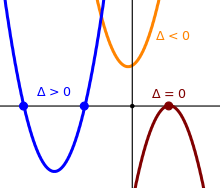Figure 3. Discriminant signs

In the quadratic formula, the expression underneath the square root sign is called the discriminant of the quadratic equation, and is often represented using an upper case D or an upper case Greek delta:A quadratic equation with real coefficients can have either one or two distinct real roots, or two distinct complex roots. In this case the discriminant determines the number and nature of the roots. There are three cases:

• If the discriminant is positive, then there are two distinct rootsboth of which are real numbers. For quadratic equations with rational coefficients, if the discriminant is a square number, then the roots are rational—in other cases they may be quadratic irrationals.
• If the discriminant is zero, then there is exactly one real rootsometimes called a repeated or double root.
• If the discriminant is negative, then there are no real roots. Rather, there are two distinct (non-real) complex rootswhich are complex conjugates of each other. In these expressions i is the imaginary unit.

Thus the roots are distinct if and only if the discriminant is non-zero, and the roots are real if and only if the discriminant is non-negative.

### Geometric interpretation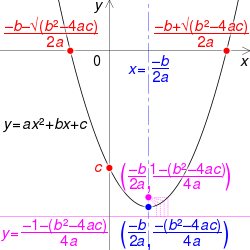Graph of y = ax² + bx + c, where a and the discriminant (b² - 4ac) are positive, with
• Roots and y-intercept in red
• Vertex and axis of symmetry in blue
• Focus and directrix in pink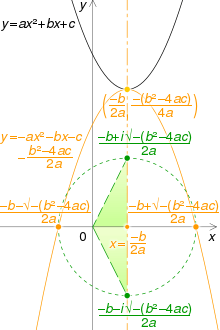Visualisation of the complex roots of y = ax² + bx + c : the parabola is rotated 180° about its vertex (yellow). Its x-intercepts are rotated 90° around their mid-point, and the Cartesian plane is interpreted as the complex plane (green).

The function f(x) = ax2 + bx + c is the quadratic function. The graph of any quadratic function has the same general shape, which is called a parabola. The location and size of the parabola, and how it opens, depend on the values of a, b, and c. As shown in Figure 1, if a > 0, the parabola has a minimum point and opens upward. If a < 0, the parabola has a maximum point and opens downward. The extreme point of the parabola, whether minimum or maximum, corresponds to its vertex. The x-coordinate of the vertex will be located at, and the y-coordinate of the vertex may be found by substituting this x-value into the function. The y-intercept is located at the point (0, c).

The solutions of the quadratic equation ax2 + bx + c = 0 correspond to the roots of the function f(x) = ax2 + bx + c, since they are the values of x for which f(x) = 0. As shown in Figure 2, if a, b, and c are real numbers and the domain of f is the set of real numbers, then the roots of f are exactly the x-coordinates of the points where the graph touches the x-axis. As shown in Figure 3, if the discriminant is positive, the graph touches the x-axis at two points; if zero, the graph touches at one point; and if negative, the graph does not touch the x-axis.

The termis a factor of the polynomialif and only if r is a root of the quadratic equationIt follows from the quadratic formula thatIn the special case b2 = 4ac where the quadratic has only one distinct root (i.e. the discriminant is zero), the quadratic polynomial can be factored as### Graphing for real roots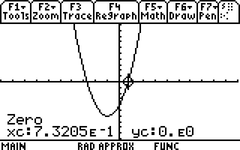Figure 4. Graphing calculator computation of one of the two roots of the quadratic equation 2x2 + 4x 4 = 0. Although the display shows only five significant figures of accuracy, the retrieved value of xc is 0.732050807569, accurate to twelve significant figures.

For most of the 20th century, graphing was rarely mentioned as a method for solving quadratic equations in high school or college algebra texts. Students learned to solve quadratic equations by factoring, completing the square, and applying the quadratic formula. Recently, graphing calculators have become common in schools and graphical methods have started to appear in textbooks, but they are generally not highly emphasized.

Being able to use a graphing calculator to solve a quadratic equation requires the ability to produce a graph of y = f(x), the ability to scale the graph appropriately to the dimensions of the graphing surface, and the recognition that when f(x) = 0, x is a solution to the equation. The skills required to solve a quadratic equation on a calculator are in fact applicable to finding the real roots of any arbitrary function.

Since an arbitrary function may cross the x-axis at multiple points, graphing calculators generally require one to identify the desired root by positioning a cursor at a "guessed" value for the root. (Some graphing calculators require bracketing the root on both sides of the zero.) The calculator then proceeds, by an iterative algorithm, to refine the estimated position of the root to the limit of calculator accuracy.

### Avoiding loss of significance

Although the quadratic formula provides an exact solution, the result is not exact if real numbers are approximated during the computation, as usual in numerical analysis, where real numbers are approximated by floating point numbers (called "reals" in many programming languages). In this context, the quadratic formula is not completely stable.

This occurs when the roots have different order of magnitude, or, equivalently, when b2 and b2 − 4ac are close in magnitude. In this case, the subtraction of two nearly equal numbers will cause loss of significance or catastrophic cancellation in the smaller root. To avoid this, the root that is smaller in magnitude, r, can be computed aswhere R is the root that is bigger in magnitude.

A second form of cancellation can occur between the terms b2 and 4ac of the discriminant, that is when the two roots are very close. This can lead to loss of up to half of correct significant figures in the roots.

## Examples and applicationsThe trajectory of the cliff jumper is parabolic because horizontal displacement is a linear function of time, while vertical displacement is a quadratic function of time. As a result, the path follows quadratic equation, whereandare horizontal and vertical components of the original velocity, a is gravitational acceleration and h is original height. The a value should be considered negative here, as its direction (downwards) is opposite to the height measurement (upwards).

The golden ratio is found as the solution of the quadratic equationThe equations of the circle and the other conic sectionsellipses, parabolas, and hyperbolasare quadratic equations in two variables.

Given the cosine or sine of an angle, finding the cosine or sine of the angle that is half as large involves solving a quadratic equation.

The process of simplifying expressions involving the square root of an expression involving the square root of another expression involves finding the two solutions of a quadratic equation.

Descartes' theorem states that for every four kissing (mutually tangent) circles, their radii satisfy a particular quadratic equation.

The equation given by Fuss' theorem, giving the relation among the radius of a bicentric quadrilateral's inscribed circle, the radius of its circumscribed circle, and the distance between the centers of those circles, can be expressed as a quadratic equation for which the distance between the two circles' centers in terms of their radii is one of the solutions. The other solution of the same equation in terms of the relevant radii gives the distance between the circumscribed circle's center and the center of the excircle of an ex-tangential quadrilateral.

## History

Babylonian mathematicians, as early as 2000 BC (displayed on Old Babylonian clay tablets) could solve problems relating the areas and sides of rectangles. There is evidence dating this algorithm as far back as the Third Dynasty of Ur. In modern notation, the problems typically involved solving a pair of simultaneous equations of the form:which are equivalent to the equation::86The steps given by Babylonian scribes for solving the above rectangle problem were as follows:

1. Compute half of p.
2. Square the result.
3. Subtract q.
4. Find the square root using a table of squares.
5. Add together the results of steps (1) and (4) to give x. This is essentially equivalent to calculatingGeometric methods were used to solve quadratic equations in Babylonia, Egypt, Greece, China, and India. The Egyptian Berlin Papyrus, dating back to the Middle Kingdom (2050 BC to 1650 BC), contains the solution to a two-term quadratic equation. In the Indian Sulba Sutras, circa 8th century BC, quadratic equations of the form ax2 = c and ax2 + bx = c were explored using geometric methods. Babylonian mathematicians from circa 400 BC and Chinese mathematicians from circa 200 BC used geometric methods of dissection to solve quadratic equations with positive roots. Rules for quadratic equations were given in the The Nine Chapters on the Mathematical Art, a Chinese treatise on mathematics. These early geometric methods do not appear to have had a general formula. Euclid, the Greek mathematician, produced a more abstract geometrical method around 300 BC. With a purely geometric approach Pythagoras and Euclid created a general procedure to find solutions of the quadratic equation. In his work Arithmetica, the Greek mathematician Diophantus solved the quadratic equation, but giving only one root, even when both roots were positive.

In 628 AD, Brahmagupta, an Indian mathematician, gave the first explicit (although still not completely general) solution of the quadratic equation ax2 + bx = c as follows: "To the absolute number multiplied by four times the [coefficient of the] square, add the square of the [coefficient of the] middle term; the square root of the same, less the [coefficient of the] middle term, being divided by twice the [coefficient of the] square is the value." (Brahmasphutasiddhanta, Colebrook translation, 1817, page 346):87 This is equivalent to:The Bakhshali Manuscript written in India in the 7th century AD contained an algebraic formula for solving quadratic equations, as well as quadratic indeterminate equations (originally of type ax/c = y Muhammad ibn Musa al-Khwarizmi (Persia, 9th century), inspired by Brahmagupta, developed a set of formulas that worked for positive solutions. Al-Khwarizmi goes further in providing a full solution to the general quadratic equation, accepting one or two numerical answers for every quadratic equation, while providing geometric proofs in the process. He also described the method of completing the square and recognized that the discriminant must be positive,:230 which was proven by his contemporary 'Abd al-Hamīd ibn Turk (Central Asia, 9th century) who gave geometric figures to prove that if the discriminant is negative, a quadratic equation has no solution.:234 While al-Khwarizmi himself did not accept negative solutions, later Islamic mathematicians that succeeded him accepted negative solutions,:191 as well as irrational numbers as solutions. Abū Kāmil Shujā ibn Aslam (Egypt, 10th century) in particular was the first to accept irrational numbers (often in the form of a square root, cube root or fourth root) as solutions to quadratic equations or as coefficients in an equation. The 9th century Indian mathematician Sridhara wrote down rules for solving quadratic equations.

The Jewish mathematician Abraham bar Hiyya Ha-Nasi (12th century, Spain) authored the first European book to include the full solution to the general quadratic equation. His solution was largely based on Al-Khwarizmi's work. The writing of the Chinese mathematician Yang Hui (1238–1298 AD) is the first known one in which quadratic equations with negative coefficients of 'x' appear, although he attributes this to the earlier Liu Yi. By 1545 Gerolamo Cardano compiled the works related to the quadratic equations. The quadratic formula covering all cases was first obtained by Simon Stevin in 1594. In 1637 René Descartes published La Géométrie containing the quadratic formula in the form we know today. The first appearance of the general solution in the modern mathematical literature appeared in an 1896 paper by Henry Heaton.

### Alternative methods of root calculation

#### Vieta's formulas

Main article: Vieta's formulas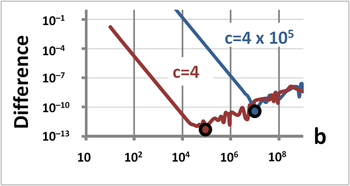Figure 5. Graph of the difference between Vieta's approximation for the smaller of the two roots of the quadratic equation x2 + bx + c = 0 compared with the value calculated using the quadratic formula. Vieta's approximation is inaccurate for small b but is accurate for large b. The direct evaluation using the quadratic formula is accurate for small b with roots of comparable value but experiences loss of significance errors for large b and widely spaced roots. The difference between Vieta's approximation versus the direct computation reaches a minimum at the large dots, and rounding causes squiggles in the curves beyond this minimum.

Vieta's formulas give a simple relation between the roots of a polynomial and its coefficients. In the case of the quadratic polynomial, they take the following form:andThese results follow immediately from the relation:which can be compared term by term withThe first formula above yields a convenient expression when graphing a quadratic function. Since the graph is symmetric with respect to a vertical line through the vertex, when there are two real roots the vertex's x-coordinate is located at the average of the roots (or intercepts). Thus the x-coordinate of the vertex is given by the expressionThe y-coordinate can be obtained by substituting the above result into the given quadratic equation, givingAs a practical matter, Vieta's formulas provide a useful method for finding the roots of a quadratic in the case where one root is much smaller than the other. If |x2| << |x1|, then x1 + x2 x1, and we have the estimate:The second Vieta's formula then provides:These formulas are much easier to evaluate than the quadratic formula under the condition of one large and one small root, because the quadratic formula evaluates the small root as the difference of two very nearly equal numbers (the case of large b), which causes round-off error in a numerical evaluation. Figure 5 shows the difference between (i) a direct evaluation using the quadratic formula (accurate when the roots are near each other in value) and (ii) an evaluation based upon the above approximation of Vieta's formulas (accurate when the roots are widely spaced). As the linear coefficient b increases, initially the quadratic formula is accurate, and the approximate formula improves in accuracy, leading to a smaller difference between the methods as b increases. However, at some point the quadratic formula begins to lose accuracy because of round off error, while the approximate method continues to improve. Consequently, the difference between the methods begins to increase as the quadratic formula becomes worse and worse.

This situation arises commonly in amplifier design, where widely separated roots are desired to ensure a stable operation (see step response).

#### Trigonometric solution

In the days before calculators, people would use mathematical tableslists of numbers showing the results of calculation with varying argumentsto simplify and speed up computation. Tables of logarithms and trigonometric functions were common in math and science textbooks. Specialized tables were published for applications such as astronomy, celestial navigation and statistics. Methods of numerical approximation existed, called prosthaphaeresis, that offered shortcuts around time-consuming operations such as multiplication and taking powers and roots. Astronomers, especially, were concerned with methods that could speed up the long series of computations involved in celestial mechanics calculations.

It is within this context that we may understand the development of means of solving quadratic equations by the aid of trigonometric substitution. Consider the following alternate form of the quadratic equation,where the sign of the ± symbol is chosen so that a and c may both be positive. By substitutingand then multiplying through by cos2θ, we obtainIntroducing functions of 2θ and rearranging, we obtainwhere the subscripts n and p correspond, respectively, to the use of a negative or positive sign in equation . Substituting the two values of θn or θp found from equations  or  into  gives the required roots of . Complex roots occur in the solution based on equation  if the absolute value of sin 2θp exceeds unity. The amount of effort involved in solving quadratic equations using this mixed trigonometric and logarithmic table look-up strategy was two-thirds the effort using logarithmic tables alone. Calculating complex roots would require using a different trigonometric form.

To illustrate, let us assume we had available seven-place logarithm and trigonometric tables, and wished to solve the following to six-significant-figure accuracy:1. A seven-place lookup table might have only 100,000 entries, and computing intermediate results to seven places would generally require interpolation between adjacent entries.
2.3.4.5.6.7.(rounded to six significant figures)#### Solution for complex roots in polar coordinates

If the quadratic equationwith real coefficients has two complex rootsthe case whererequiring a and c to have the same sign as each otherthen the solutions for the roots can be expressed in polar form aswhereand#### Geometric solution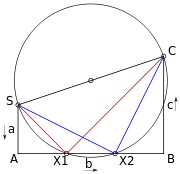Figure 6. Geometric solution of ax2 + bx + c = 0 using Lill's method. Solutions are −AX1/SA, −AX2/SA

The quadratic equation may be solved geometrically in a number of ways. One way is via Lill's method. The three coefficients a, b, c are drawn with right angles between them as in SA, AB, and BC in Figure 6. A circle is drawn with the start and end point SC as a diameter. If this cuts the middle line AB of the three then the equation has a solution, and the solutions are given by negative of the distance along this line from A divided by the first coefficient a or SA. If a is 1 the coefficients may be read off directly. Thus the solutions in the diagram are AX1/SA and AX2/SA.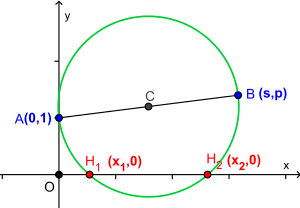Carlyle circle of the quadratic equation x2  sx + p = 0.

The Carlyle circle, named after Thomas Carlyle, has the property that the solutions of the quadratic equation are the horizontal coordinates of the intersections of the circle with the horizontal axis. Carlyle circles have been used to develop ruler-and-compass constructions of regular polygons.

The formula and its derivation remain correct if the coefficients a, b and c are complex numbers, or more generally members of any field whose characteristic is not 2. (In a field of characteristic 2, the element 2a is zero and it is impossible to divide by it.)

The symbolin the formula should be understood as "either of the two elements whose square is b2 4ac, if such elements exist". In some fields, some elements have no square roots and some have two; only zero has just one square root, except in fields of characteristic 2. Even if a field does not contain a square root of some number, there is always a quadratic extension field which does, so the quadratic formula will always make sense as a formula in that extension field.

#### Characteristic 2

In a field of characteristic 2, the quadratic formula, which relies on 2 being a unit, does not hold. Consider the monic quadratic polynomialover a field of characteristic 2. If b = 0, then the solution reduces to extracting a square root, so the solution isand there is only one root sinceIn summary,In the case that b 0, there are two distinct roots, but if the polynomial is irreducible, they cannot be expressed in terms of square roots of numbers in the coefficient field. Instead, define the 2-root R(c) of c to be a root of the polynomial x2 + x + c, an element of the splitting field of that polynomial. One verifies that R(c) + 1 is also a root. In terms of the 2-root operation, the two roots of the (non-monic) quadratic ax2 + bx + c areandFor example, let a denote a multiplicative generator of the group of units of F4, the Galois field of order four (thus a and a + 1 are roots of x2 + x + 1 over F4. Because (a + 1)2 = a, a + 1 is the unique solution of the quadratic equation x2 + a = 0. On the other hand, the polynomial x2 + ax + 1 is irreducible over F4, but it splits over F16, where it has the two roots ab and ab + a, where b is a root of x2 + x + a in F16.

This is a special case of Artin–Schreier theory.

1. Protters & Morrey: " Calculus and Analytic Geometry. First Course"
2. Washington, Allyn J. (2000). Basic Technical Mathematics with Calculus, Seventh Edition. Addison Wesley Longman, Inc. ISBN 0-201-35666-X.
3. Ebbinghaus, Heinz-Dieter; Ewing, John H. (1991), Numbers, Graduate Texts in Mathematics, 123, Springer, p. 77, ISBN 9780387974972.
4. Sterling, Mary Jane (2010), Algebra I For Dummies, Wiley Publishing, p. 219, ISBN 978-0-470-55964-2
5. Rich, Barnett; Schmidt, Philip (2004), Schaum's Outline of Theory and Problems of Elementary Algebra, The McGraw-Hill Companies, ISBN 0-07-141083-X, Chapter 13 §4.4, p. 291
6. Himonas, Alex. Calculus for Business and Social Sciences, p. 64 (Richard Dennis Publications, 2001).
7. Kahan, Willian (November 20, 2004), On the Cost of Floating-Point Computation Without Extra-Precise Arithmetic (PDF), retrieved 2012-12-25
8. Alenit͡syn, Aleksandr and Butikov, Evgeniĭ. Concise Handbook of Mathematics and Physics, p. 38 (CRC Press 1997)
9. Δ is the initial of the Greek word Διακρίνουσα, Diakrínousa, discriminant.
10. Achatz, Thomas; Anderson, John G.; McKenzie, Kathleen (2005). Technical Shop Mathematics. Industrial Press. p. 277. ISBN 0-8311-3086-5.
11. "Complex Roots Made Visible -- Math Fun Facts". Retrieved 1 October 2016.
12. Wharton, P. (2006). Essentials of Edexcel Gcse Math/Higher. Lonsdale. p. 63. ISBN 978-1-905-129-78-2.
13. Ballew, Pat. "Solving Quadratic Equations By analytic and graphic methods; Including several methods you may never have seen" (PDF). Retrieved 18 April 2013.
14. Higham, Nicholas (2002), Accuracy and Stability of Numerical Algorithms (2nd ed.), SIAM, p. 10, ISBN 978-0-89871-521-7
15. Friberg, Jöran (2009). "A Geometric Algorithm with Solutions to Quadratic Equations in a Sumerian Juridical Document from Ur III Umma". Cuneiform Digital Library Journal. 3.
16. Stillwell, John (2004). Mathematics and Its History (2nd ed.). Springer. ISBN 0-387-95336-1.
17. The Cambridge Ancient History Part 2 Early History of the Middle East. Cambridge University Press. 1971. p. 530. ISBN 978-0-521-07791-0.
18. Henderson, David W. "Geometric Solutions of Quadratic and Cubic Equations". Mathematics Department, Cornell University. Retrieved 28 April 2013.
19. Aitken, Wayne. "A Chinese Classic: The Nine Chapters" (PDF). Mathematics Department, California State University. Retrieved 28 April 2013.
20. Smith, David Eugene (1958). History of Mathematics. Courier Dover Publications. p. 380. ISBN 978-0-486-20430-7.
21. Smith, David Eugene (1958). History of Mathematics, Volume 1. Courier Dover Publications. p. 134. ISBN 0-486-20429-4. Extract of page 134
22. Katz, V. J.; Barton, B. (2006). "Stages in the History of Algebra with Implications for Teaching". Educational Studies in Mathematics. 66 (2): 185201. doi:10.1007/s10649-006-9023-7.
23. Boyer, Carl B.; Uta C. Merzbach, rev. editor (1991). A History of Mathematics. John Wiley & Sons, Inc. ISBN 0-471-54397-7.
24. O'Connor, John J.; Robertson, Edmund F., "Arabic mathematics: forgotten brilliance?", MacTutor History of Mathematics archive, University of St Andrews. "Algebra was a unifying theory which allowed rational numbers, irrational numbers, geometrical magnitudes, etc., to all be treated as "algebraic objects"."
25. Jacques Sesiano, "Islamic mathematics", p. 148, in Selin, Helaine; D'Ambrosio, Ubiratan, eds. (2000), Mathematics Across Cultures: The History of Non-Western Mathematics, Springer, ISBN 1-4020-0260-2
26. Smith, David Eugene (1958). History of Mathematics. Courier Dover Publications. p. 280. ISBN 978-0-486-20429-1.
27. Livio, Mario (2006). The Equation that Couldn't Be Solved. Simon & Schuster. ISBN 0743258215.
28. Ronan, Colin (1985). The Shorter Science and Civilisation in China. Cambridge University Press. p. 15. ISBN 978-0-521-31536-4.
29. Struik, D. J.; Stevin, Simon (1958), The Principal Works of Simon Stevin, Mathematics (PDF), II–B, C. V. Swets & Zeitlinger, p. 470
30. Heaton, H (1896). "A Method of Solving Quadratic Equations". American Mathematical Monthly. 3 (10): 236–237. doi:10.2307/2971099. JSTOR 2971099.
31. Seares, F. H. (1945). "Trigonometric Solution of the Quadratic Equation". Publications of the Astronomical Society of the Pacific. 57 (339): 307309. Bibcode:1945PASP...57..307S. doi:10.1086/125759.
32. Aude, H. T. R. (1938). "The Solutions of the Quadratic Equation Obtained by the Aid of the Trigonometry". National Mathematics Magazine. 13 (3): 118121. doi:10.2307/3028750. JSTOR 3028750.
33. Simons, Stuart, "Alternative approach to complex roots of real quadratic equations", Mathematical Gazette 93, March 2009, 91–92.
34. Bixby, William Herbert (1879), Graphical Method for finding readily the Real Roots of Numerical Equations of Any Degree, West Point N. Y.
35. Weisstein, Eric W. "Carlyle Circle". From MathWorld—A Wolfram Web Resource. Retrieved 21 May 2013.Wikimedia Commons has media related to Quadratic equation.